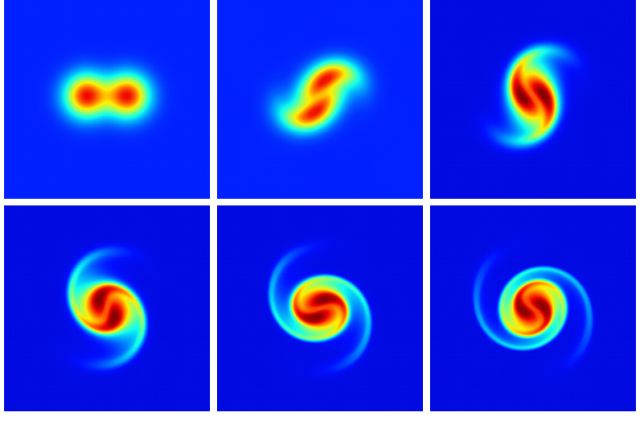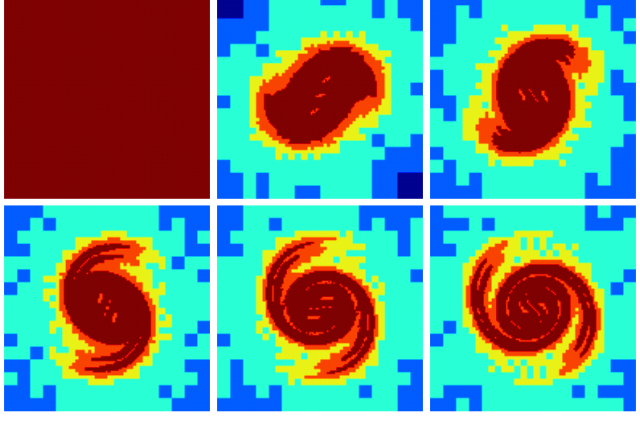# Merging of two vortices (centered Euler solver)

This test is similar to stream.c but uses the centered Navier–Stokes solver (without viscosity). It also shows how to convert a vorticity field into a velocity field.

#include "navier-stokes/centered.h"

The domain is centered on (0,0) and the maximum level of refinement is 8 i.e. the initial grid has 2^8=256 grid points per dimension.

#define MAXLEVEL 8

// This is necessary for convergence when lowering the tolerance
uf.n[left]   = 0.;
uf.n[right]  = 0.;
uf.n[top]    = 0.;
uf.n[bottom] = 0.;

int main()
{
origin (-0.5, -0.5);
init_grid (1 << MAXLEVEL);
//  TOLERANCE = 1e-12;
run();
}

For the centered Navier–Stokes solver, the primary variables are the velocity and pressure field. We need to convert the initial vorticity field into the velocity field. To do so we first declare the (temporary) streamfunction \psi and vorticity \omega fields. We also set appropriate boundary conditions for the streamfunction.

event init (t = 0)
{
scalar psi[], omega[];

psi[left]   = dirichlet(0);
psi[right]  = dirichlet(0);
psi[top]    = dirichlet(0);
psi[bottom] = dirichlet(0);

We then initialise both fields, using the same initial condition for the vorticity as in stream.c, and apply boundary conditions. Note that it is necessary to initialise the streamfunction, as the Poisson solver requires an initial guess.

  double dd = 0.1;
foreach() {
omega[] = (exp(-(sq(x - dd) + sq(y))/(dd/10.)) +
exp(-(sq(x + dd) + sq(y))/(dd/10.)));
psi[] = 0.;
}
boundary ({psi,omega});

We then solve the Poisson equation \displaystyle \nabla^2\psi = \omega and compute the centered velocity components by differentation of the streamfunction i.e. \displaystyle u_x = - \partial_y\psi \displaystyle u_y = \partial_x\psi

  poisson (psi, omega);
coord f = {-1.,1.};
foreach()
foreach_dimension()
u.x[] = f.x*(psi[0,1] - psi[0,-1])/(2.*Delta);
boundary ((scalar *){u});
}

We output some statistics on the vorticity field and Poisson solver at the start and end of the simulation.

event logfile (t <= 30; t += 1) {
scalar omega[];
vorticity (u, omega);
stats s = statsf (omega);
fprintf (stderr, "%g %d %g %g %g %d\n", t, i, dt, s.sum, s.max, mgp.i);
}

We make animations of the vorticity and level of refinement.

event movie (t += 0.2; t <= 30) {
scalar omega[];
vorticity (u, omega);
output_ppm (omega, linear = true, file = "vort.mp4");

foreach()
omega[] = level;
output_ppm (omega, spread = 2, file = "level.mp4");

foreach()
omega[] = pid();
output_ppm (omega, min = 0, max = npe() - 1, file = "pid.mp4");
}

We output the vorticity and level fields at regular intervals in a format compatible with gnuplot.

event output (t += 5) {
static int nf = 0;
scalar omega[];
vorticity (u, omega);

char name;
sprintf (name, "omega-%d", nf);
FILE * fp = fopen (name, "w");
output_field ({omega}, fp, linear = true);
fclose (fp);

scalar l = omega;
foreach()
l[] = level;
sprintf (name, "level-%d", nf);
fp = fopen (name, "w");
output_field ({l}, fp);
fclose (fp);
nf++;
}

If we are using a tree grid, it is adapted using wavelet error control on both components of the velocity field. Note that the error thresholds need to be specified twice (once for each component of vector \mathbf{u}).

#if TREE
}
#endif

## Results

After running and processing by gnuplot we get the following pictures and animations.

set term @PNG enhanced size 640,426
set output 'vorticity.png'
set size ratio -1
unset key
unset xtics
unset ytics
unset border
unset colorbox
set pm3d
set pm3d map interpolate 1,1
set palette defined ( 0 0 0 0.5647, 0.125 0 0.05882 1, 0.25 0 0.5647 1, \
0.375 0.05882 1 0.9333, 0.5 0.5647 1 0.4392, \
0.625 1 0.9333 0, 0.75 1 0.4392 0, \
0.875 0.9333 0 0, 1 0.498 0 0 )

set multiplot layout 2,3 scale 1.6,1.6
splot 'omega-0'
splot 'omega-1'
splot 'omega-2'
splot 'omega-3'
splot 'omega-4'
splot 'omega-5'
unset multiplotset output 'level.png'
set cbrange [3:8]
set multiplot layout 2,3 scale 1.6,1.6
splot 'level-0'
splot 'level-1'
splot 'level-2'
splot 'level-3'
splot 'level-4'
splot 'level-5'
unset multiplot